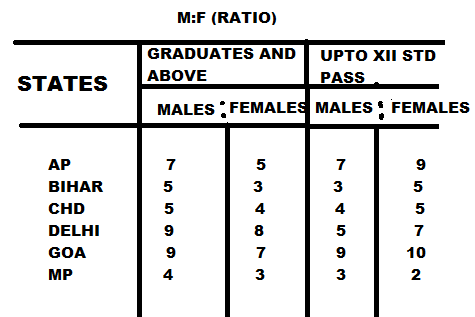New Students Offer - Use Code HELLO

# Data Interpretation Practice Set - Double Diagram (Part 2)

Study the following pie chart and table to answer these questions.1) What is the difference between the graduate male population and XII Std male population from AP ?
(a) 24000
(b) 14000
(c) 28000
(d) 36000
(e) None of these

2) What is the ratio of female population of Goa to XII Std female population of Delhi respectively?
(a) 7:5
(b) 5:7
(c) 16:15
(d) 15:16
(e) None of these

3) Graduate female population of Chandigarh what per cent of the XII Std female population of the state ?
(a) 40%
(b) 62.5%
(c) 50 %
(d) 52.5%
(e) None of these

4) Class XII pass male population of Chandigarh is what per cent of the total XII Std population of all the states together ?
(a) 8%
(b) 12%
(c) 11%
(d) 9%
(e) None of these

5) What is the ratio of graduation male population of the Goa to XII Std female population of that state ?
(a) 28:35
(b) 35:28
(c) 32:45
(d) 45:32
(e) None of these

6) Total graduate population of state MP is what per cent of the total XII Std population of AP ?
(a) 56%
(b) 72%
(c) 68%
(d) 72%
(e) None of these

7) XII Std male population of Goa is what per cent of XII Std male population of MP?
(a) 70%
(b) 75%
(c) 68%
(d) 72%
(e) None of these

8) What is the ratio of the total graduate and XII Std male population of AP to the total graduate and XII Std of AP to the total graduate and XII Std female population of that state ?
(a) 215:216
(b) 214:215
(c) 217:215
(d) 215:217
(e) None of these

9) What is the ratio of the total graduate population of the Delhi to the total Std population of that state?
(a) 17:16
(b) 16:17
(c) 64:51
(d) 51:64
(e)None of these

10) Graduate female population of Bihar is what per cent of the graduate female population of Goa ? (rounded off to nearest integer)
(a) 129%
(b) 82%
(c) 77%
(d) 107%
(e) None of these

(1) b
(2) d
(3) c
(4) a
(5) e
(6) e
(7) b
(8) c
(9) a
(10) c

### SOLUTIONS

1) Graduate male population of AP = (24  *16 / 100 * 7/12) lakh = 2.24 lakh
XII Std male population of AP = (32  *12 / 100 * 7 / 16) lakh = 2.1 lakh

=> Required difference = (2.24 - 2.1) lakh = 14000

2) Graduate female population of Goa = (24 * 20 / 100 * 7 / 16) lakh = 2.1 lakh
XII Std female population of Delhi = (32 * 12 / 100 * 7 / 12) lakh = 2.24 lakh

=> Required Ration = 2.1:2.24 = 210:224 = 15:16

3) Graduate female population of Chandigarh = (24 * 15/100 *4/9) lakh = 1.6 lakh
XII Std female population of Chandigarh = (32 * 18/100 * 5/9) lakh =3.2 l lakh

=> Required percentage = 1.6/3.2 * 100 = 50%

4) XII Std male population of Chandigarh = 32 * 18/100 * 4/9 = 2.56 lakh

=> Required percentage = 2.56/32 * 100 = 8%

5) Graduate male population of Goa  = 24 * 20/100 * 9/16 = 2.7 lakh
XII Std female population of Goa = 32 * 19/100 * 10/19 = 3.2 lakh

=> Required Ratio = 27:32

6) Total graduate population of MP = 24 * 14/100 = 3.36 lakh
XII Std total population of AP = 32 * 15/100 = 4.8 lakh

=> Required Percentage = 3.36/4.8 * 100 = 70%

7) XII Std pass male population of Goa = 32 * 19/100 * 9/19 = 2.88 lakh
XII Std pass male population of MP = 32 * 20/100 * 3/5 = 3.84 lakh

=> Required Percentage = 2.88/3.84 * 100 = 75%

8) Graduate male population of AP = 24 * 7/12 * 6/100 =2.24 lakh
XII Std pass male population of state AP = 32 * 15/100 * 7/16 = 2.1 lakh
Sum = (2.24 + 2.1) lakh = 4.34 lakh

Graduate female population of AP = 24 * 5/12 16/100 = 1.6 lakh
XII Std pass female population of state AP = 32 * 15/100 * 9/16 = 2.7 lakh
Sum = (1.6 + 2.7) = 4.3 lakh

=> Required ratio = 434:430 = 217:215

9) Total population of Delhi = 17% of 24 lakh
Total XII Std population of Delhi = 12% of 32 lakh

=> Required Percentage = 17% of 24 lakh : 12% of 32 lakh
= 17*24 : 12*32
=17 : 16

10) Graduate female population of Bihar = 24 * 18/100 * 3/8 = 1.62 lakh
Graduate female population of Goa = 24 * 20/100 * 7/16 = 2.1 lakh

=> Required Percentage = 1.62/2.1 * 100 = 77%